# Schrödinger's wave equation

(redirected from Schrodinger's wave equation)
Also found in: Dictionary, Thesaurus.

## Schrödinger's wave equation

A linear, homogeneous partial differential equation that determines the evolution with time of a quantum-mechanical wave function.

Quantum mechanics was developed in the 1920s along two different lines, by W. Heisenberg and by E. Schrödinger. Schrödinger's approach can be traced to the notion of wave-particle duality that flowed from A. Einstein's association of particlelike energy bundles (photons, as they were later called) with electromagnetic radiation, which, classically, is a wavelike phenomenon. For radiation of definite frequency f, each bundle carries energy hf. The proportionality factor, h = 6.626 × 10-34 joule-second, is a fundamental constant of nature, introduced by M. Planck in his empirical fit to the spectrum of blackbody radiation. This notion of wave-particle duality was extended in 1923 by L. de Broglie, who postulated the existence of wavelike phenomena associated with material particles such as electrons. See Photon, Wave mechanics

There are certain purely mathematical similarities between classical particle dynamics and the so-called geometric optics approximation to propagation of electromagnetic signals in material media. For the case of a single (nonrelativistic) particle moving in a potential V( r ), this analogy leads to the association with the system of a wave function, &PSgr;( r ), which obeys

(1)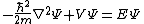Eq. (1). Here m is the mass of the particle, E its energy, &planck; = h/(2&pgr;), and ∇2 is the laplacian operator. See Geometrical optics

It is possible to ask what more general equation a time- as well as space-dependent wave function, &PSgr;( r , t), might obey. What suggests itself is Eq. (2),

(2)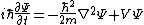which is now called the Schrödinger equation.

The wave function can be generalized to a system of more than one particle, say N of them. A separate wave function is not assigned to each particle. Instead, there is a single wave function, &PSgr;( r1, r2, …, rN , t), which depends at once on all the position coordinates as well as time. This space of position variables is the so-called configuration space. The generalized Schrödinger equation is Eq. (3),

(3)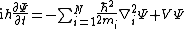where the potential V may now depend on all the position variables. Three striking features of this equation are to be noted:

1. The complex number i (the square root of minus one) appears in the equation. Thus &PSgr; is in general complex.

2. The time derivative is of first order. Thus, if the wave function is known as a function of the position variables at any one instant, it is fully determined for all later times.

3. The Schrödinger equation is linear and homogeneous in &PSgr;, which means that if &PSgr; is a solution so is c&PSgr;, where c is an arbitrary complex constant. More generally, if &PSgr;1 and &PSgr;2 are solutions, so too is the linear combination c1&PSgr;1 + c2&PSgr;2, where c1 and c2 are arbitrary complex constants. This is the superposition principle of quantum mechanics. See Superposition principle

The Schrödinger equation suggests an interpretation in terms of probabilities. Provided that the wave function is square integrable over configuration space, it follows from Eq. (3) that the norm, 〈&PSgr;&PSgr;〉, is independent of time, where the norm is defined by Eq. (4). (4)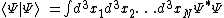It is possible to normalize &PSgr; (multiply it by a suitable constant) to arrange that this norm is equal to unity. With that done, the Schrödinger equation itself suggests that expression (5)

(5)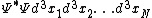is the joint probability distribution at time t for finding particle 1 in the volume element d3x1, particle 2 in d3x2, and so forth.

Site: Follow: Share:
Open / Close